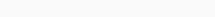# Math Puzzles Puzzle 57 Answer AndroidMath puzzle game level 57 solution with detailed hints and explanation, Scroll below to find out.

Math puzzles increase your logical thinking. The objective of the game is to find patterns hidden in the puzzle in order to arrive at the solution. Challenge yourself with different levels of math puzzles and stretch the limits of your intelligence.

### Math Puzzles Puzzle 57:

if 1,3=2

4,9=5

5,11=6

7,32=9

then 10,21=?